# Part 13 of the basics of 4D complex numbers: Factorization of the determinant.

It is about high time for a small update. Originally I wanted to include a little rant against all those math professors that have stated that the so called Euler formulae for the exponential circle in the complex plane is the most beautiful piece of math ever.

How can you say that and after all those other exponential circles and curves I found stay silent year in year out? We now have a fresh academic year and likely the new year nothing will happen again.

The same goes for magnetism, if it is true electrons carry magnetic charge one way or the other this will have huge economical impacts in the long run. Not only can you better understand how spintronic devices can work but as a negative also understand how nuclear fusion with those Tokomak things will never work because of the electron acceleration.

In my view this is important given the speed of climate change and how slow we react on it while at the same time we are always promised golden mountains of almost free energy if only we had nuclear fusion…

But likely university people are university people so we will just observe one more academic year of just nothing.

__________

After having said that, in this post we calculate the determinant via multiplying the four eigenvalues every 4D complex number Z has. Of course that is always when such a Z is viewed as it’s matrix representation M(Z).

You can do much more with that kind of stuff, in the previous part number 12 we unearthed the eigenvalues and eigenvectors so we can also do the nice thing of matrix diagonalization. I have not planned anything about the content in the next few parts of these small series on 4D complex numbers. So we’ll see.

This post is five pictures long, at the end I also show you the so called cylinder equation from 3D space (circular version).

Have fun reading it and thinking about it a little bit. See you around.

Before the actual post I will show you the teaser picture as published on the other website, it contains the matrix who’s determinant we are going to factor. When I read all these posts on 4D complex numbers backwards it was only in the basics number 3 where I mentioned this explicit matrix representation M(Z).

In the teaser picture you also see the main result. I never worked out the determinant of the matrix M(Z) via a method like expansion via the minors or so. Just going straightforward for the eigenvalue functions and multiply these in order to get the determinant for any 4D complex number Z.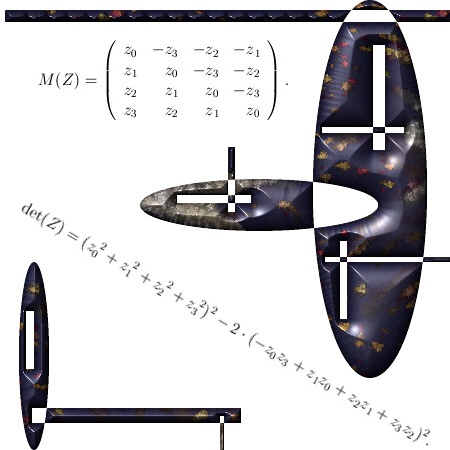So it is the determinant of the matrix representation M(Z) we are going to factorize.
Here we go: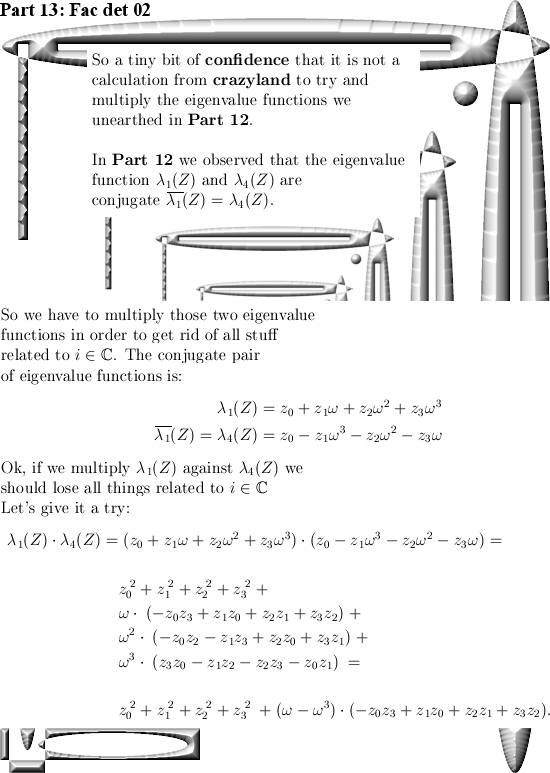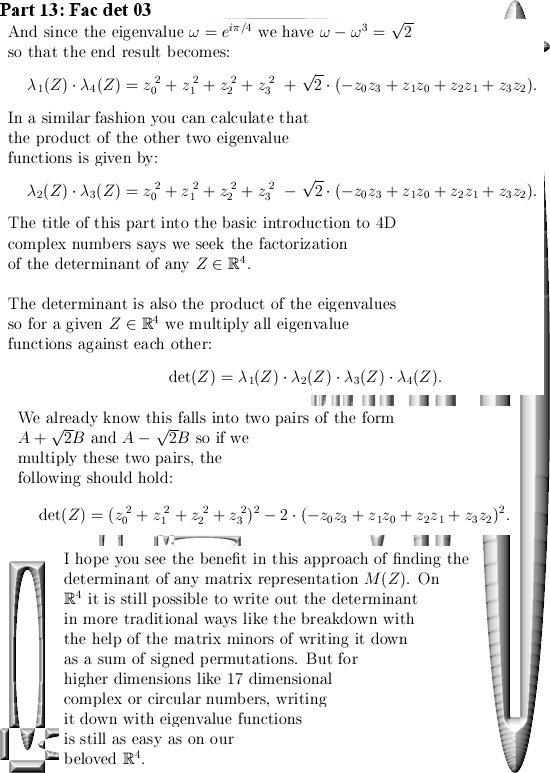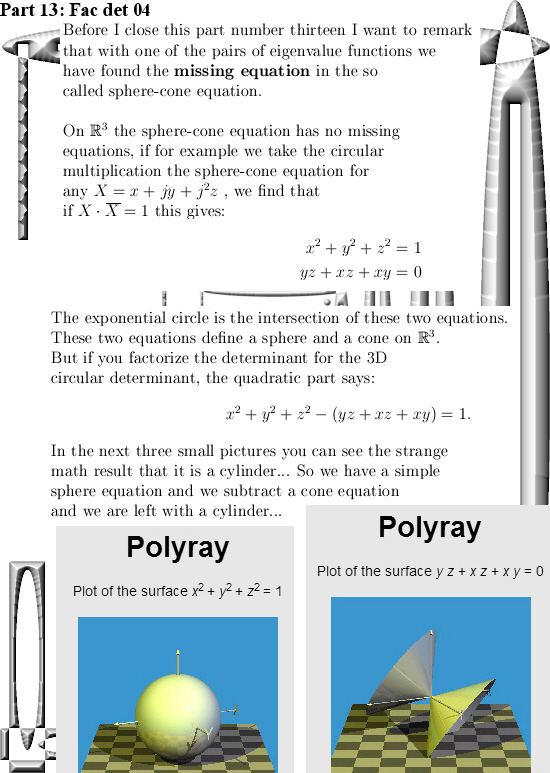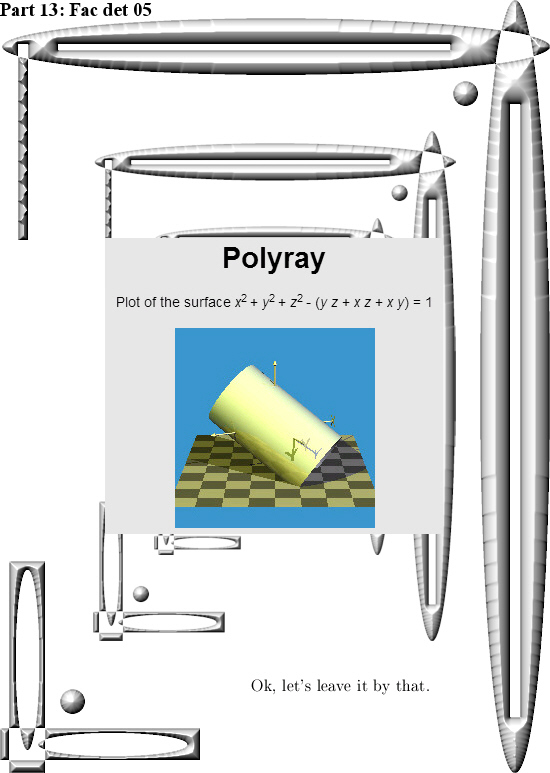Ok, let´s not rant because why waste all that emotional energy on university people?
But it is now almost your years back since I crafted a pdf about the first 10 exponential circles and curves I found:

An overview of exponential circles and curves in …
http://kinkytshirts.nl/pdfs/10_exponential_circles_and_curves.pdf

That was from 22 December 2014. We can safely conclude that at most universities a lot is happening, but it is mostly weird stuff. Weird stuff like ‘The sum of all integers equals minus 1/12…’ Oh oh, if you have people like that inside your ranks how can that bring any good?

So is it science or comedy in the next video?

It is more like comedy I just guess…

But what would life be without comedy? That would also be a strange place to live, a life without observable comedians likely is a less funny place to live in.

Hey let’s pop open one more pint of beer. By the way in the quantum world every thing is different and at first I could not believe that, why would that be? It was years and years later I found out that quantum particles like electrons never drink beer. Just never. And at that point in time I finally understood just how different the quantum world is compared to our human world.

# Part 12 of the 4D complex numbers: The four eigenvalue functions for a arbitrary 4D complex number.

In Part 11 we found the eigenvalues and eigenvectors of the first imaginary unit l (with of course the property l^4 = -1). But if we have those eigenvalues for l,it is easy to find the eigenvectors of powers of l.

But every 4D complex number is a sum of a real part and the three imaginary parts with the units  l,  l ^2 and l ^3. So from a linear algebra point of view it is also easy to find the eigenvalues of such combinations of  l. Needless to say that in this post you should read  l and it powers mostly as it’s matrix representation M(l).

I have to admit that I very often avoid the constant need for the matrix representation, for example I mostly write det(l) for the determinant of M(l). But it makes texts just so unreadable if you constant write det(M(l)), I do not like that.

When you understand how to calculate the eigenvalue functions as shown below, please remark it looks a like the so called discrete Fourier transform.

This post is five pictures long (each 550×775 pixels) although the last picture is rather empty… And why no an empty picture? After all when you had a paper book in the good old days, there were always empty pages in it. Sometimes it was even written that ‘This page is left empty intentionally’. And in those long lost years that was the crime of the century because if you write on a page that it is empty, that is never true…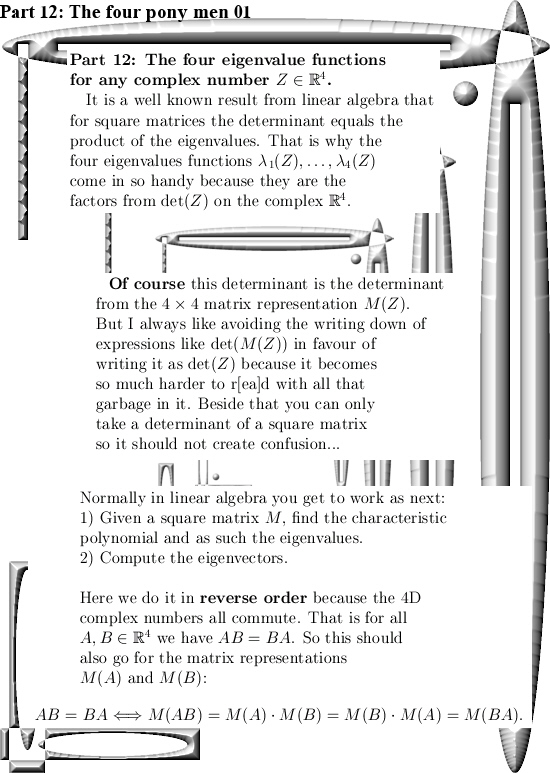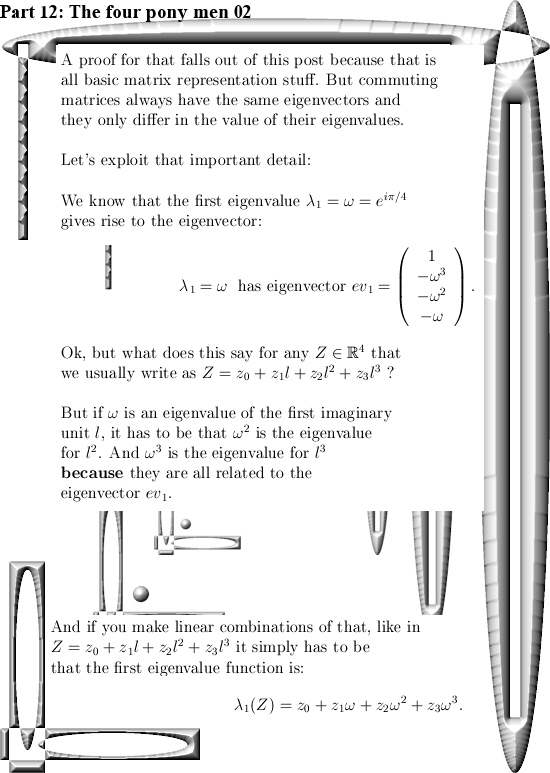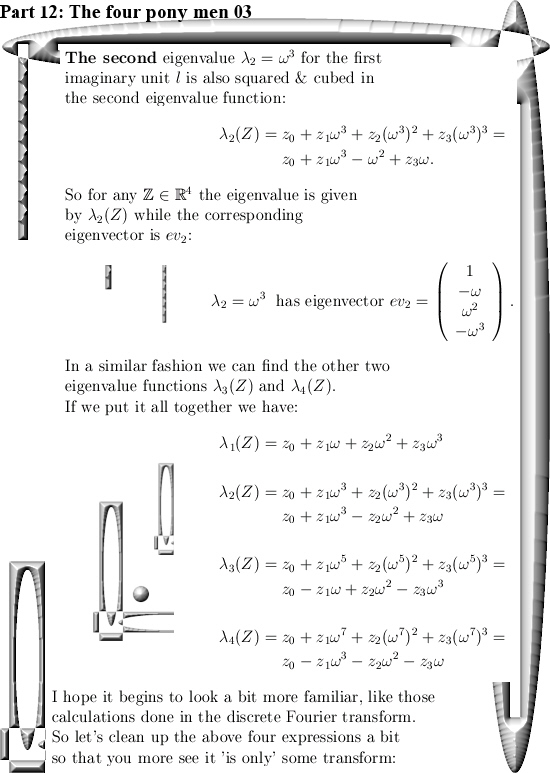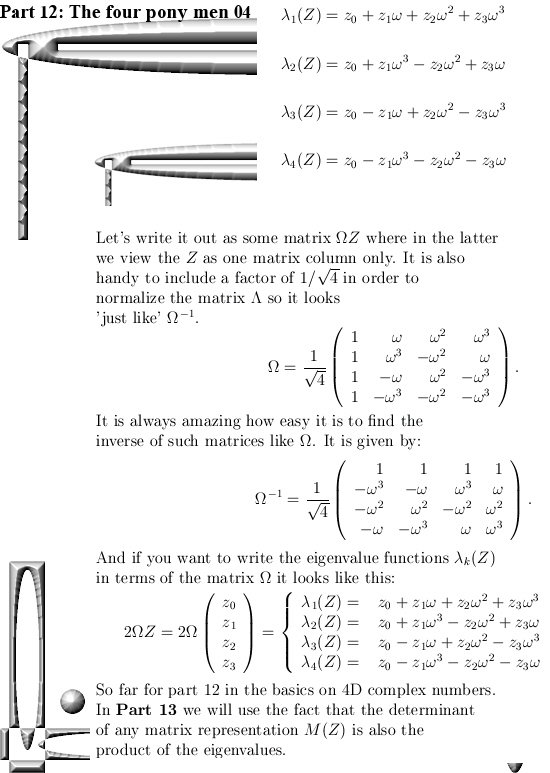In the next post we will take a look at the missing equation we still have when it comes to the calculation of the 4D exponential curve when it comes to the  sphere/cone equation. we missed one equation to arrive at a 1D solution for our exponential curve.

If you also include the demand that the determinant of the exponential curve is 1 all of the time, you can squeeze out more equations inside the 4D complex numbers.

Before we say goodbye, here is a link to the matrix representation of the discrete Fourier transform. But take you time when thinking about matrices like in the link or the Omega matrix as above.

DFT matrix
https://en.wikipedia.org/wiki/DFT_matrix

Ok, that was it. Till updates.